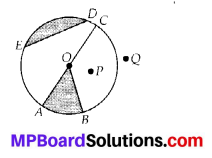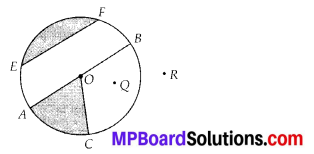## MP Board Class 6th Maths Solutions Chapter 4 Basic Geometrical Ideas Ex 4.6

Question 1.
From the figure, identify:(a) the centre of circle
(c) a diameter
(d) a chord
(e) two points in the interior
(f) a point in the exterior
(g) a sector
(h) a segment
Solution:
(a) O is the centre of circle.
(b) Three radii: $$\overline{O A}$$, $$\overline{O B}$$ and $$\overline{O C}$$
(c) A diameter : $$\overline{A C}$$
(d) A chord : $$\overline{E D}$$
(e) Two interior points : O and P
(f) Exterior point: Q
(g) A sector : OAB (shaded part)
(h) A segment: ED (shaded part)Question 2.
(a) Is every diameter of a circle also a chord?
(b) Is every chord of a circle also a diameter?
Solution:
(a) Yes, every diameter of a circle is also a chord. It is the largest chord of a circle.
(b) No, every chord of a circle is not a diameter.Question 3.
Draw any circle and mark
(a) its centre
(c) a diameter
(d) a sector
(e) a segment
(f) a point in its interior
(g) a point in its exterior
Solution:(a) O is the centre of the circle.
(b) $$\overline{O A}$$ is the radius
(c) $$\overline{A B}$$ is the diameter.
(d) OAC is the sector, (shaded part)
(e) EF is the segment, (shaded part)
(f) Q is a point in its interior.
(g) R is a point in its exterior.
(h) $$\widehat{A C}$$ is an arc.Question 4.
Say true or false:
(a) Two diameters of a circle will necessarily intersect.
(b) The centre of a circle is always in its interior.
Solution:
(a) True
(b) True